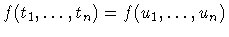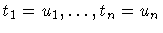Next: Structured Data and Data Up: Adding Computation Domains Previous: Herbrand Terms

# Herbrand Terms: Syntactic Equality

Terms can only be equated between them. The only constraint allowed is =/2, representing syntactic equality. Two terms are equated by binding variables to subterms so that the initial terms become equal. The formal algorithm which does that is:

• An equationis solved by solving.
• An equation v = t, where v is a variable and t is a term which does not contain v is solved with the binding v/t (in other words, v = t is already solved, since this represents a variable which is equated to a term not containing that variable).
• Equations like t = t can be deleted.
• If none of the above can be applied, the initial terms cannot be unified.

The algorithm is written in terms of equality equations to emphasize the fact that Herbrand terms are just another kind of constraint system. Below are some examples of equating terms; those examples have been directly taken from the toplevel of a CLP interpreter:

```?- X = f(a, b).
X = f(a, b)
```

Equating X with f(a,b) is made by just binding X (a previously free variable) to f(a,b).

```?- X = f(T, a), T = b.
T = b, X = f(b,a)
```

In this case T is bound to b; in turn T appears in the term f(T, a), which is equated to X. After performing all possible substitutions of variables, X becomes bound to f(b, a)

```?- f(X, g(X, Y), Y) = f(a, T, b).
X = a, Y = b, T = g(a, b)
```

In this example we try to equate two terms. Both have the same toplevel functor (f/3), so that the subterms in corresponding argument positions are compared one by one, and all the resulting equations solved. First, X = a because of the first argument. Then, T = g(X, Y), but, since X = a previously, in fact the equation generated is T = g(a, Y). In the last argument, Y = b. As Y appeared in a previous equation, this one is rewritten to T = g(a, b). The final result is a system of equations in solved form: there are only variables at the left hand side of the equations, and none of them appear at the right hand side of the equations. This can be viewed as a binding of variables to terms. Note: choosing left hand side or right hand side is not important: the equations can be rotated.Next: Structured Data and Data Up: Adding Computation Domains Previous: Herbrand Terms
MCL
1998-12-03